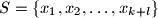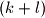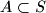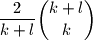### IMO Shortlist 2008 problem C5

Kvaliteta:
Avg: 0,0
Težina:
Avg: 8,0
Let$S = \{x_1, x_2, \ldots, x_{k + l}\}$ be a$(k + l)$-element set of real numbers contained in the interval$[0, 1]$;$k$ and$l$ are positive integers. A$k$-element subset$A\subset S$ is called nice ifProve that the number of nice subsets is at least$\dfrac{2}{k + l}\dbinom{k + l}{k}$.